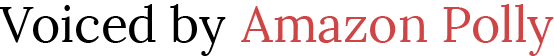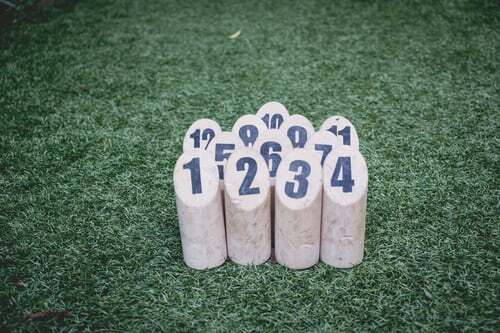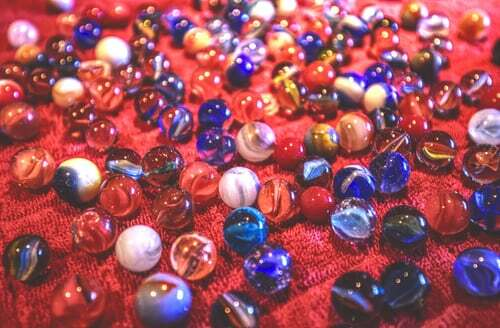# Teaching Properties of Numbers to 7th Graders

 Would You Rather Listen to the Lesson?Properties of real numbers is one of the earliest lessons students learn in pre-algebra in 7th grade. By using the properties of numbers, 7th graders are prepared to easily simplify expressions and solve linear equations.

To help students achieve mastery of properties of real numbers, math teachers can benefit from a number of tips. Read on to learn more about such tips that are guaranteed to keep your students engaged throughout the lesson!## What Are Properties of Numbers (7th Grade)?

For starters, you can explain to children how we define properties of real numbers. Properties of real numbers are simply “laws” of numbers that apply to certain math operations. Point out that there are four basic properties:

• Commutative
• Associative
• Identity
• Distributive

Make sure to highlight that these properties don’t apply to all operations, but only when we add or multiply numbers. In other words, the properties of real numbers don’t work for division or subtraction. You can illustrate this later with examples as you go through each property.

## How to Teach Properties of Numbers (7th Grade)

After providing a brief introduction, you can provide an overview of each property separately, alongside examples of how it’s used. You can also rely on using videos to teach this lesson in your class, such as this fun video that features a ‘Mathemagician’ showing the properties of real numbers by using magical math tricks.

### Commutative Property

The commutative property states that we can change the order of the numbers that we’re adding, but it won’t affect the answer. That is, we can swap out numbers but the sum remains the same. We can present this in the following way:

a + b = b + a

Provide a few examples, such as:

3 + 2 = 2 + 3 = 5

4 + 5 = 5 + 4 = 9

A useful way of demonstrating this is with the help of marbles. Bring a few marbles to class and

share them between two student groups – group one should have four marbles and group two should have five marbles. Let children visualize the commutative property by swapping the groups and counting the sum of marbles, which stays the same even when the groups are swapped.#### Multiplication

The commutative property also holds true for multiplications, stating that we can swap over the numbers that are being multiplied without making any difference to the product. That is, regardless of the order of numbers being multiplied, the product remains the same.

We can present this in the following way:

a x b = b x a

Provide a few examples, such as:

4 x 2 = 2 x 4 = 8

3 x 5 = 5 x 3 = 15

#### Subtraction and Division

Finally, remind children that the commutative property doesn’t work when we’re dividing or subtracting numbers. Demonstrate this with a few examples, such as:

20 ÷ 4 = 5

But:

4 ÷ 20 = 0.2

5 – 1 = 4

But:

1 – 5 = – 4

### Associative Property

Explain that the associative property refers to number grouping, that is, which one we calculate first. It states that when adding three or more numbers, we can change the way in which we group these numbers, but this won’t affect the sum. We can present it like this:

(a + b) + c = a + (b + c)

Provide an example, such as:

(2 + 3) + 5 = 2 + (3 + 5) = 10

#### Multiplication

Add that the associative property also applies to multiplication. It states that when we’re multiplying three or more numbers, we can change the grouping of the numbers being multiplied, but this won’t affect the product. We can present it like this:

(a × b) × c = a × (b × c)

Provide an example, such as:

(2 x 3) x 5 = 2 x (3 x 5) = 30

#### Subtraction and Division

Same as with the commutative property, the associative property does not work for subtraction or division. Demonstrate this with an example, such as:

(8 ÷ 4) ÷ 2 = 1

But:

8 ÷ (4 ÷ 2) = 4

### Identity Property

Explain that the identity property states that for any number a, the sum of 0 and the number a is the number itself. That is, by adding any real number to zero, the sum is equal to the number itself. We can present this property for addition in the following way:

a + 0 = a

0 + a = a

Provide a few examples to demonstrate how this works, for instance:

– 4 + 0 = – 4

And also:

0 + (-4) = – 4

3 + 0 = 3

And also:

0 + 3 = 3

#### Multiplication

Add that the identity property also works for multiplication. That is, the identity property states that for any number a, the product of 1 and the number a is the number itself. We can present this in the following way:

a x 1 = a

Provide a few examples, such as:

7 x 1 = 7

– 23 x 1 = – 23

### Distributive Property

As the name suggests, the distributive property helps us distribute a given expression. This property states that if we add two or more numbers and multiply this sum to an outside number, – the result will be the same if we multiply every number in the parenthesis by the outside number and then add the products.

We can present this in the following way:

a x (b + c) = a x b + a x c

Provide an example of this, such as:

3 x (4 + 5) = 3 x 4 + 3 x 5 = 27

## Activities to Practice Properties of Numbers (7th Grade)

### Group Work

Use this simple activity at the end of the lesson to reinforce students’ knowledge of the properties of real numbers. To prepare for this activity, simply print out this Assignment Worksheet. Make sure you make enough copies for each student.

The worksheet contains exercises with equations where children are asked to evaluate an expression and then identify the type of property that is used in each equation. Writing and evaluating expressions is something that children should already master, but you can refresh their skills by referring to this article.

Divide children into groups of 3, 4. Hand out the worksheets and provide instructions. All members of a given group work together to solve the exercises. In the end, each group presents their answers in front of the class.

If there’s time left, you can also use this Assignment Worksheet that focuses on solving math problems by applying the distributive property of numbers. As these exercises are more advanced, you can leave them for the end to provide a challenge to students.

Parents who are homeschooling their children can easily transform this activity into an individual activity. Or if you have an older child, you can simply involve them in reviewing the properties of numbers!

## Before You Leave…

If you liked these tips on teaching properties of numbers to 7th graders, we have a whole free lesson that’s dedicated to this topic! So make sure you check out our worksheets and resources below. These are all PDFs, so they’ll print out easily:

To get the Editable versions of these files, join us in the Math Teacher Coach community!